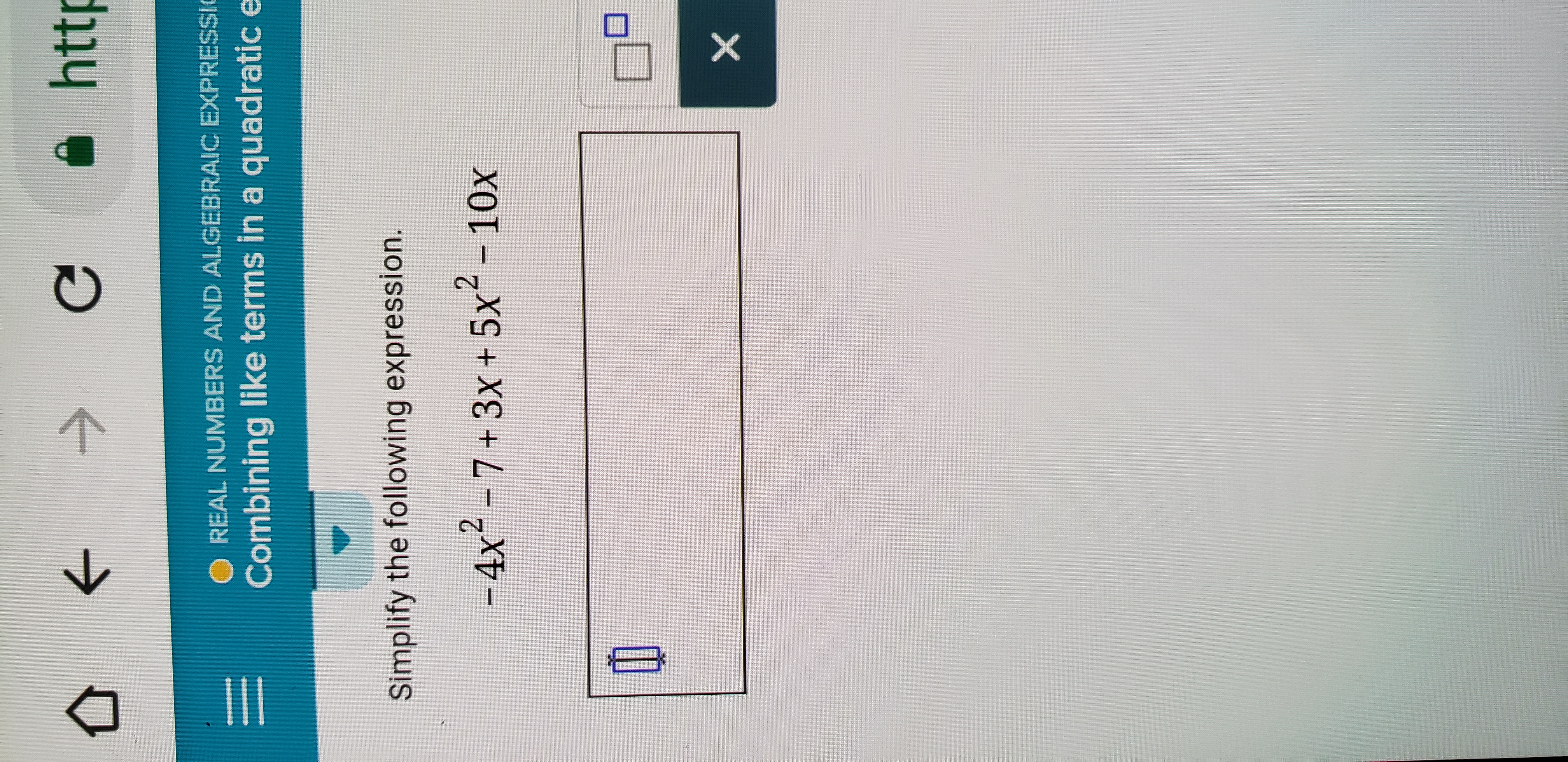公←→-O REAL NUMBERS AND ALGEBRAIC EXPRESSİCombining like terms in a quadratic eSimplify the following expression.-4x2 -7+3x +5x2 -10x

Questionhelp_outlineImage Transcriptionclose公←→ -O REAL NUMBERS AND ALGEBRAIC EXPRESSİ Combining like terms in a quadratic e Simplify the following expression. -4x2 -7+3x +5x2 -10x fullscreen
Step 1

We have been given an algebraic expression and we need to simplify it.

Step 2

In order to simplify the given expression, we will first collect the like terms and combine them. Like terms are those terms which contain the same variable of same degree. For ( an independent ) example, 2a an...

Want to see the full answer?

See Solution

Want to see this answer and more?

Our solutions are written by experts, many with advanced degrees, and available 24/7

See Solution
Tagged in

Equations and In-equations